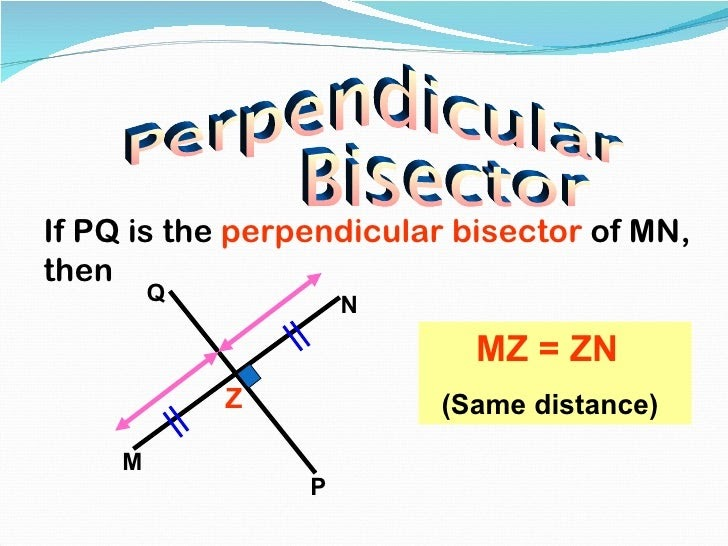# Perpendicular Bisector Calculator

Enter Values in the given input box to find the Perpendicular bisector equation using our Perpendicular bisector calculator.

## Perpendicular bisector definition

Perpendicular Bisector is the division of something into two equal or congruent parts. It is a line, ray, or segment which cuts another line segment into two equal parts at 90 degrees.

The perpendicular bisector equation can be effortlessly calculated using the perpendicular bisector calculator. Constructing a perpendicular bisector could be convenient if you know how to use a compass?

## Perpendicular bisector equation Formula

y-y1 = m(x-x1)

Input Data :
Coordinates 1 (x1, y1) = (2, 3)
Coordinates 2 (x2, y2) = (8, 7)

Objective :
Find what is the perpendicular bisector of a line?

Formula :
y – y1 = m (x – x1)
x1 & y1 are midpoint of the co-ordinates
m is slope of the line

Solution :
Midpoint of the straight line
Midpoint =(x1 + x22 y1 + y22)
=(2 + 82 3 + 72)
=(102 102)
Midpoint = (5, 5)

Find what is the slope of given line
Slope =y2 – y1x2 – x1
=7 – (3)8 – (2)
=46

Find the negative reciprocal as follows
m =-14/6
m = -1.5

Use the values to arrange perpendicular bisector equation
y – y1 = m (x – x1)
y – 5 = -1.5 (x – 5)
y – 5 = -1.5x + 7.5
y = -1.5x + 7.5 + 5
y = -1.5x + 12.5

### Hex to Decimal Converter## Perpendicular Bisector Calculator

Perpendicular Bisector Calculator is an online tool for geometry calculation programmed to find out the perpendicular bisector of a line according to the given coordinates (x1, y1) and (x2, y2). In Geometry, a perpendicular bisector is a group of points that are equidistant from coordinates (x1, y1) and (x2, y2). The shape of the group always forms a line. Any point on the perpendicular bisector is as far from coordinates (x1, y1) as from coordinates (x2, y2). The given line coordinates (x1, y1) and (x2, y2) in the XY plane are used in this calculator to find out the perpendicular bisector of a line. Govt Jobs